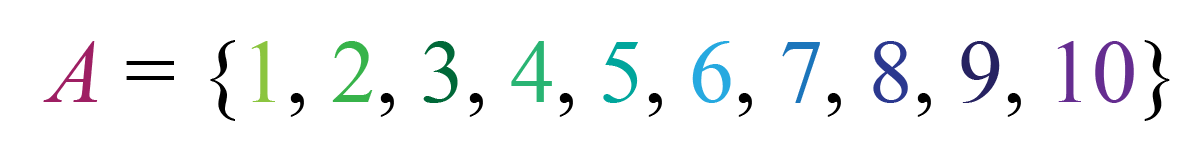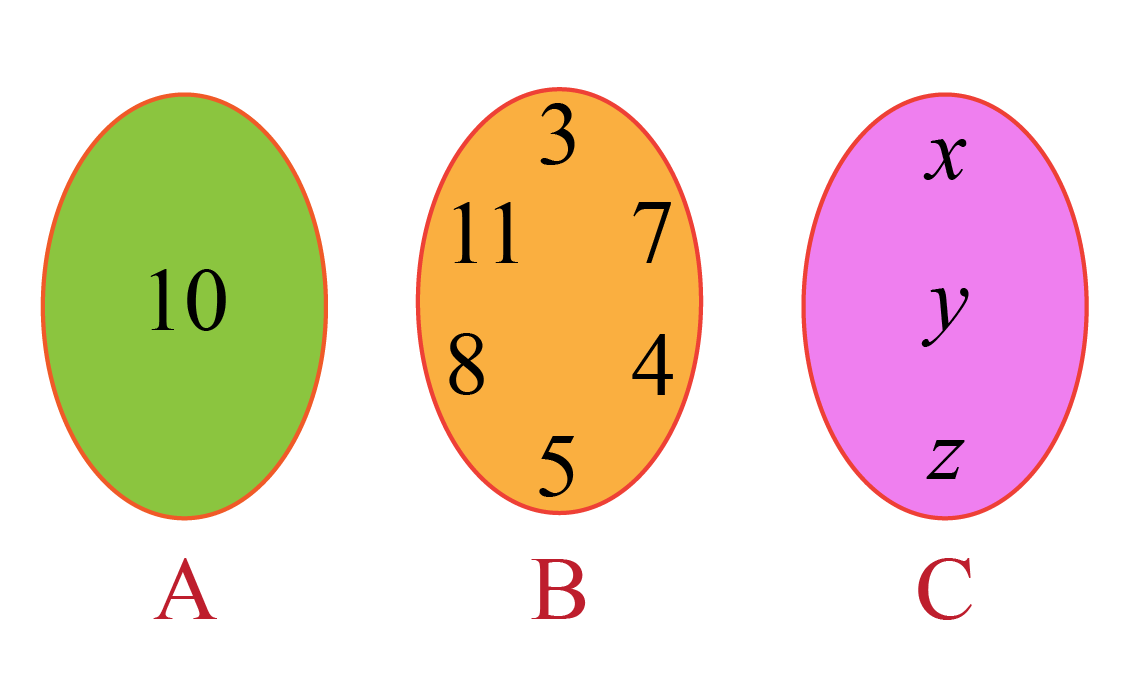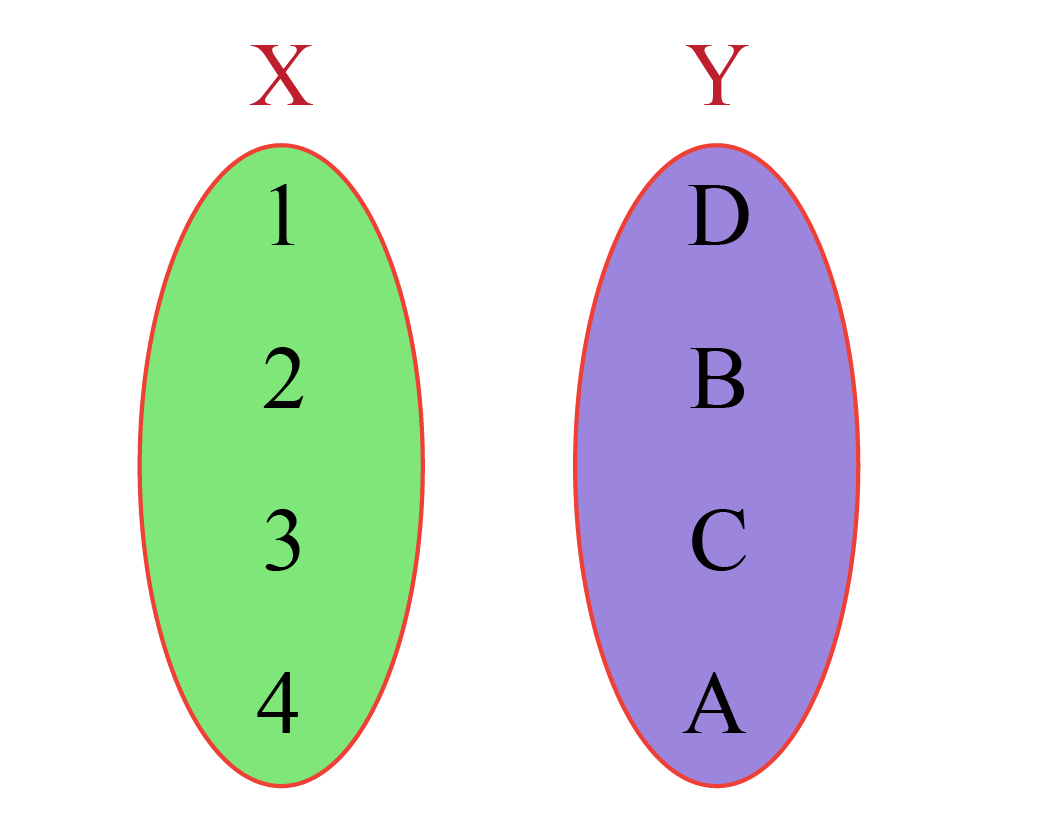# Roster Notation

Roster Notation

In this mini-lesson, we will learn about roster notation by understanding the use of roster notation, different types of numbers used in roster notation, and how to apply them while solving problems. We will also discover interesting facts around them.

We all have a habit of arranging our things in a proper manner.

We don't put clothes in a bookshelf nor do we put shoes in a wardrobe. Everything at home has a specific use.We arrange things to identify them easily.

We have a similar concept in mathematics where we arrange a particular set of numbers together.

As the name suggests, the term is called sets. A number of sets can be represented by four notations - one of which is called roster notation.

In this mini-lesson, we will learn more about roster notations.

## Lesson Plan

 1 What Is Meant by Roster Notation? 2 Thinking Out of the Box! 3 Solved Examples on Roster Notation 4 Important Notes on Roster Notation 5 Interactive Questions on Roster Notation

## What Is Meant by Roster Notation?

Before getting into the details of roster notations, let us understand the foundation of sets better.

Sets

If we can clearly define and decide the objects of a given collection, then that collection is called a set.

Generally, we use capital letters to name the set A, B, C,....., Z

The contents or the elements of the set are denoted by using small letters a, b, c, ...

If a is an element of set A, then we write it as $$‘a \in A'$$ and if a is not an element of set A then we write $$‘a \notin A'$$.

Sets can be represented in different forms. The four common forms of representing them are.

• Set-builder notation
• Roster notation
• Interval notation
• graphical notation

Roster Notation

In roster notation, the elements of a set are represented in a row surrounded by curly brackets and if the set contains more than one element then every two elements are separated by commas.

For example, if A is the set of first 10 natural numbers so it can be represented by:## Why Do We Use Roster Notation?

Roster notation is one of the most simple techniques to represent the elements of a set.

The elements can be represented in a row and are easy to read and understand.

For example, the set of the first 20 natural numbers divisible by 5 can be represented in roster notation like:

A = {5, 10, 15, 20, 25, 30, 35, 40, 45, 50, 55, 60, 65, 70, 75, 80, 85, 90, 95, 100}

The dotted line at the end represents the continuation of the elements of the set, it means that the numbers after 25 are not shown but it is the part of the set A.Set A contains only a single element hence the elements can be represented with curly brackets.

$\text{A = {10}}$

Set B contains multiple elements hence the elements can be represented as:

$\text{B = {3, 7, 4, 5, 8, 11}}$

The elements of set C can be represented as:

$\text{C = {x, y, z}}$

One of the limitations of roster notation is that we cannot represent a large number of data in roster notation.

For example, if we want to represent the first 100 or 200 natural numbers in a set B then it is hard for us to represent this much data in a single row.

This limitation can be overcome by representing data with the help of a dotted line.

Take a set of the first 100 positive odd numbers.

$\text{B = {1, 3, 5, 7........199}}$

The dotted line shows that the numbers are part of set B but not written in roster notation.

When we represent large numbers of elements in a set using roster notation we usually write the first few elements and the last element and we separate these elements with a comma.

Let's take a set of all the English alphabets, it can be represented like:

$\text{C = {a, b, c, d.......z}}$

If any set has infinite numbers of elements like the set of all the even positive numbers, it can be represented like:

$\text{D = {2, 4, 6, 8......}}$

We simply can denote the rest of the numbers with a dotted line since there is no end to positive even numbers we have to keep it like this.

## What Are the Symbol of Different Types of Number Used in Roster Notation?

There are different types of symbols that we use while representing the elements of any set.

These symbols can be used to represent the elements in any type of set notations.

Let's explore a few of these.

 Symbol Meaning $$\in$$ Is an element of $$\not \in$$ Is not an element of $$\mathbb{N}$$ Set of natural numbers $$\mathbb{C}$$ Set of complex numbers $$\mathbb{Z}$$ Set of integers $$\mathbb{Q}$$ Set of rational numbers $$\mathbb{R}$$ Set of real numbers $$\subset$$ Is a proper subset of $$\subseteq$$ Is a subset of $$\supset$$ Is a proper superset of $$\supseteq$$ Is a superset of $$\cup$$ Set union $$cap$$ Set intersectionThink Tank
1. Can you express the birthdays of your friends in roster notation?
2. Express the numbers of the Fibonacci series in roster notation.

## Solved Examples on Roster Notation

 Example 1

Express the below two sets X and Y in the roster notations.Solution

The set X in roster notation can be expressed like:

$\text{X = {1, 2, 3, 4}}$

The set Y in roster notation can be expressed like:

$\text{Y = {D, B, C, A}}$

 $$\therefore \text{X = {1, 2, 3, 4}}$$  $\text{Y = {D, B, C, A}}$
 Example 2

Express the set $$\text{A = {x | x = 2n^2 - 1, where n \in \mathbb{N} and n<5$$ in roster notation.

Solution

The elements of set A is:

for n = 1
\begin{align}2n^2 - 1 &= 2 \times 1^2 - 1 \\[0.2cm] &= 1\end{align}

for n = 2
\begin{align}2n^2 - 1 &= 2 \times 2^2 - 1 \\[0.2cm] &= 7\end{align}

for n = 3
\begin{align}2n^2 - 1 &= 2 \times 3^2 - 1 \\[0.2cm] &= 17\end{align}

for n = 4
\begin{align}2n^2 - 1 &= 2 \times 4^2 - 1 \\[0.2cm] &= 31\end{align}

for n = 5
\begin{align}2n^2 - 1 &= 2 \times 5^2 - 1 \\[0.2cm] &= 49\end{align}

$\text{A = {1, 7, 17, 31, 49}}$

 $$\therefore \text{A = {1, 7, 17, 31, 49}}$$
More Important Topics
Numbers
Algebra
Geometry
Measurement
Money
Data
Trigonometry
Calculus